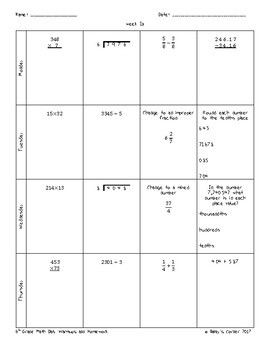# 6th Grade Math Weekly Warmups and Homework Sample6th - 7th, Homeschool
Subjects
Standards
Resource Type
Formats Included
• PDF
Pages
13 pages

### Description

This product includes a 5 week sample of my 6th Grade Math Warmups and Homework for 36 weeks. Answer key is included. Each page (side a) includes 4 warm up problems for Monday through Thursday and (side b) 4 aligned homework problems for Monday through Thursday. The warm ups are designed to be completed in class and gone over with the teacher. This gives students the opportunity to check their work and ask questions if they need to. Similar problems are then assigned for homework on the back. All 6th grade math common core standards will be reviewed.

Check out my bundles:

6th Grade Math Warmups and Homework

6th Grade Math Complete Interactive Math Notebook

Unit 1: Fraction and Decimal Operations

Unit 2: Ratios and Proportional Relationships

Unit 3: Properties and Expressions

Unit 4: Equations and Inequalities

Unit 5: Rational Numbers

Unit 6: Geometry

Unit 7: Statistics

Don't forget to rate this product to earn your TpT credits. Follow my store and my blog for updates on freebies and sales. Thank you! :)

Total Pages
13 pages
N/A
Teaching Duration
N/A
Report this Resource to TpT
Reported resources will be reviewed by our team. Report this resource to let us know if this resource violates TpT’s content guidelines.

### Standards

to see state-specific standards (only available in the US).
Find the area of right triangles, other triangles, special quadrilaterals, and polygons by composing into rectangles or decomposing into triangles and other shapes; apply these techniques in the context of solving real-world and mathematical problems.
Find the volume of a right rectangular prism with fractional edge lengths by packing it with unit cubes of the appropriate unit fraction edge lengths, and show that the volume is the same as would be found by multiplying the edge lengths of the prism. Apply the formulas 𝘝 = 𝘭 𝘸 𝘩 and 𝘝 = 𝘣 𝘩 to find volumes of right rectangular prisms with fractional edge lengths in the context of solving real-world and mathematical problems.
Draw polygons in the coordinate plane given coordinates for the vertices; use coordinates to find the length of a side joining points with the same first coordinate or the same second coordinate. Apply these techniques in the context of solving real-world and mathematical problems.
Represent three-dimensional figures using nets made up of rectangles and triangles, and use the nets to find the surface area of these figures. Apply these techniques in the context of solving real-world and mathematical problems.
Recognize a statistical question as one that anticipates variability in the data related to the question and accounts for it in the answers. For example, “How old am I?” is not a statistical question, but “How old are the students in my school?” is a statistical question because one anticipates variability in students’ ages.